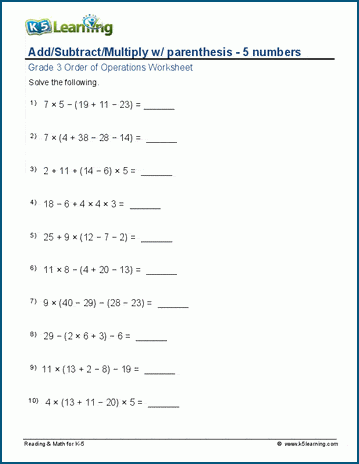# Order of operations: Add / subtract / multiply 5 numbers

## Order of Operations Worksheets: Add / subtract / multiply with parenthesis (5 numbers)

Below are six versions of our grade 3 math worksheet on adding, subtracting and multiplying 5 numbers with parenthesis.  Students need to follow the correct order of operations.  These worksheets are pdf files.## More order of operations worksheets

Browse all of our order of operations worksheets, from adding and subtracting with parenthesis to full PEMDAS worksheets including exponents.

## What is K5?

K5 Learning offers reading and math worksheets, workbooks and an online reading and math program for kids in kindergarten to grade 5.  We help your children build good study habits and excel in school.